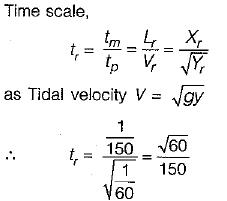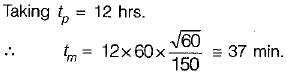Courses

# Test: Dimensional & Model Analysis - 2

## 10 Questions MCQ Test Topicwise Question Bank for Civil Engineering | Test: Dimensional & Model Analysis - 2

Description
This mock test of Test: Dimensional & Model Analysis - 2 for Civil Engineering (CE) helps you for every Civil Engineering (CE) entrance exam. This contains 10 Multiple Choice Questions for Civil Engineering (CE) Test: Dimensional & Model Analysis - 2 (mcq) to study with solutions a complete question bank. The solved questions answers in this Test: Dimensional & Model Analysis - 2 quiz give you a good mix of easy questions and tough questions. Civil Engineering (CE) students definitely take this Test: Dimensional & Model Analysis - 2 exercise for a better result in the exam. You can find other Test: Dimensional & Model Analysis - 2 extra questions, long questions & short questions for Civil Engineering (CE) on EduRev as well by searching above.
QUESTION: 1

Solution:
QUESTION: 2

### Assuming that the thrust T of a propeller depends on the diameter D, speed of advance V, angular velocity ω, dynamic viscosity μ and mass density ρ, which of the following ’non -dimensional parameters can be derived ‘by dimensional analysis?

Solution:

Number of variables = 6
Number of dimensions = 3
Dimensionless parameters = 6 - 3 = 3.
Take D, V, and ρ as repeating variables.
VD/μ will not be dimensionless- number as its dimension is [M-1L3].

QUESTION: 3

### Which of the following could be a π-parameter of the function f(F, V, r, m, L) = 0 when V, r and L are taken as repeating variables?

Solution:

A π parameter is constant and has zero dimensional unit i.e. M°L°T°.

QUESTION: 4

Match List-I (Flow problem under study) with List-ll (Model law) and select the correct answer using the codes given below the lists:
List-I
1. Rise of gas bubbles in liquid
2. Flow of gas in a pipe
3. Flow over a spillway dam
4. Flight of supersonic jet

List-II
A. Euler number
B. Froude number
C. Mach number
D. Reynold’s number
E. Weber’s number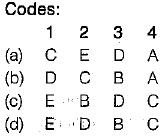Solution:
QUESTION: 5

A ship’s model of scale 1 : 100 had a wave resistance of 1 kg at its design speed. The corresponding wave resistance in prototype will be

Solution: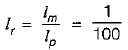Froude model is applicable here because of influence of gravity force using froude model law,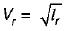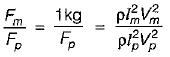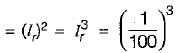or, Fp = 1000000 kg

QUESTION: 6

If the velocity, bulk modulus of elasticity and the mass density of a fluid are denoted by U, K and ρ respectively, then the Mach number is given by

Solution:

Mach number U/C =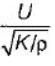QUESTION: 7

Euler’s equation of motion

Solution:

max = (fg)+ (fp)x + (fn)x + (ft)x
→ Reynold’s equation
fg → Due to gravity
fp → Due to pressure
fn → Due to viscosity
ft → Due to turbulence
max = (fg)x + (fp)x → Euler’s equation
(Neglected viscous force vand force due to turbulence)

QUESTION: 8

A model of reservoir is emptied in 10 minutes. If the model scale is 1:25, the time taken by the prototype to empty itself, would be

Solution: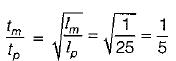∴ tp = 5tm = 5 x 10 = 50 min

QUESTION: 9

When Mach number is more than 6, the flow is called

Solution:when the Mach number in flow region is slightly less to slightly greater than 1, flow is transonic flow.

QUESTION: 10

A harbour model has a horizontal scale of 1/150 and a vertical scale of 1/60. The interval between successive high tides in the model will be nearly

Solution: